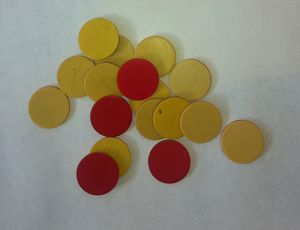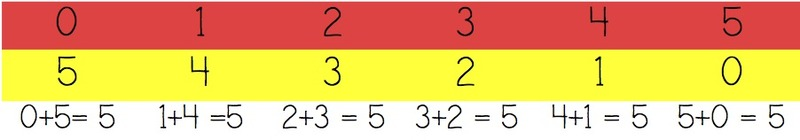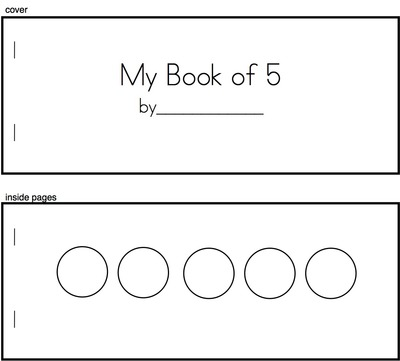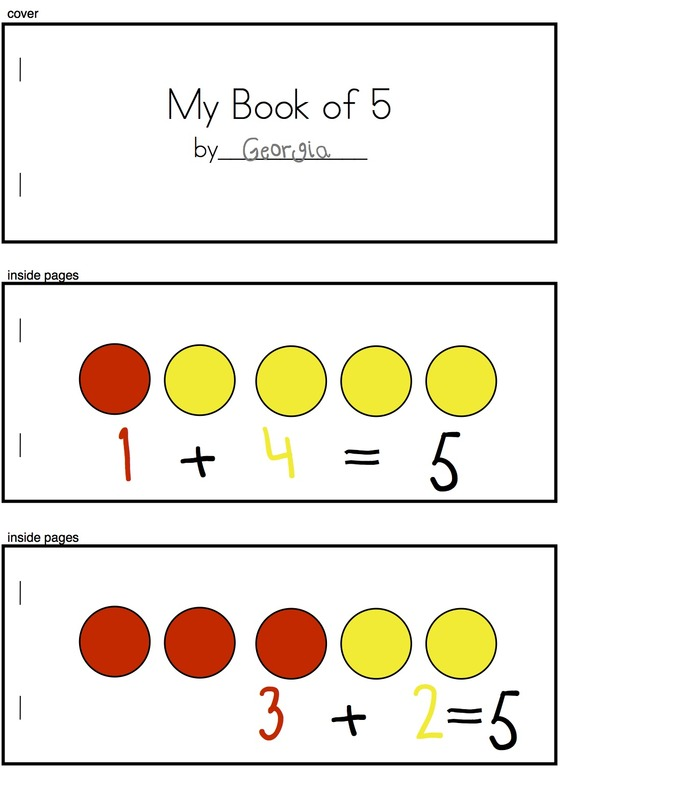# My Book of Five

Alignments to Content Standards: K.OA.A.5 K.OA.A.3

### Materials

• Double sided counters• Markers that are the same colors as the counters
• Teacher-made â€œMy Book of 5â€ (see below for detailed directions)

### Action

Students will be given double sided counters/dots (see picture of counters, above). It is important for the markers to match the colors on the counters. Students take five counters in their cupped hands (or a cup), shake them around, pour them onto the desk. Next, they count how many counters are yellow and how many are red. Students then record the numbers in their book and write a corresponding equation. For example, if the counters landed so that 1 was yellow and 4 were red, then the student would draw one yellow dot and four red dots and then write â€œ1+4=5â€ under the drawing. The student would then collect the counters and roll them again. For each combination of colors, the students record with a picture and an equation. Students continue until they fill their book of 5. The teacher can choose how many pages to put in, somewhere between five and eight is a good number so that students get a chance to see multiple combinations. After the students have completed their books, the teacher should have a whole-group discussion to make the number relationships explicit. One way to do this is to write each of the two addends into a table and to discuss possible patterns and reasons for the pattern. The teacher can ask specific questions such as, â€œWhat do you notice about the numbers in the table?â€ Or â€œWhy is it that as one number gets bigger, the other number gets smaller?â€Once students have completed the book, the activity can be repeated but with a book that has 6 pages. The students would then need to create one page for each possible way to make five. How to make "My Book of 5": The book could simply be blank pages stapled together and the student creates the circle and writes the equation under. If your students need more scaffolding each page of the book can consist of five empty circles which the students color in. This will make it impossible for the students to accidentally draw four or six circles. See below for an example: Empty Book Pages:Filled In by Student:Here is a photo of students working on different numbered books (not books of five):## IM Commentary

The purpose of this task is to help students develop fluency with addition facts to 5. The focus in developing fluency should be more than the internalization of facts but on supporting students' natural development of number sense so that students are able to solve computations flexibly and efficiently using their understanding of relationships between numbers.

Childrenâ€™s natural development of numbers progresses from the concrete to the abstract, from counting all (e.g. physically making four counters and then making twelve and counting all the counters to get sixteen), to counting on (e.g., counting four more starting at twelve to get to sixteen), to using part-whole (e.g. splitting apart the twelve to ten and two, and adding the two to four, then adding the ten) and relational thinking (knowing that 4 + 10 is 14 so 4 + 12 would be just two more).

This task supports kindergarteners at the beginning of this journey when they are counting all. It also helps students develop part-part whole thinking by having them explore all the ways that five can be made.

This task is best used multiple times with different numbers. For example teachers could have students make â€œMy Book of 5â€ as well as â€œMy Book of 4â€. Over the course of the year students can make books for numbers 4-10.

The Standards for Mathematical Practice focus on the nature of the learning experiences by attending to the thinking processes and habits of mind that students need to develop in order to attain a deep and flexible understanding of mathematics. Certain tasks lend themselves to the demonstration of specific practices by students. The practices that are observable during exploration of a task depend on how instruction unfolds in the classroom. While it is possible that tasks may be connected to several practices, only one practice connection will be discussed in depth. Possible secondary practice connections may be discussed but not in the same degree of detail.

This particular task helps illustrate Mathematical Practice Standard 8, Look for and express regularity in repeated reasoning. Students mix up five 2-colored counters and pour them onto their desks. After determining how many are red and how many are yellow, they write the corresponding equation. This process continues until all the possible equations have been written. Through questioning the teacher guides students to notice a relationship between the two addends. Â First graders use repeated reasoning and begin to notice as one addend gets bigger, the other addend gets smaller. They also may notice that the two addends are never the same. This type of investigation opens the door for making conjectures and generalizations based on repeated reasoning. As students begin to explain why these generalizations must be true, they construct, critique, and compare arguments (MP.3).

## Solution

\begin{align} 0 + 5 &= 5 \\ 1 + 4 &= 5 \\ 2 + 3 &= 5 \\ 3 + 2 &= 5 \\ 4 + 1 &= 5 \\ 5 + 0 &= 5 \end{align}